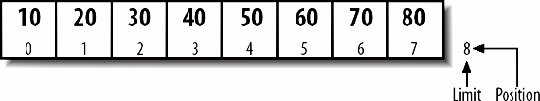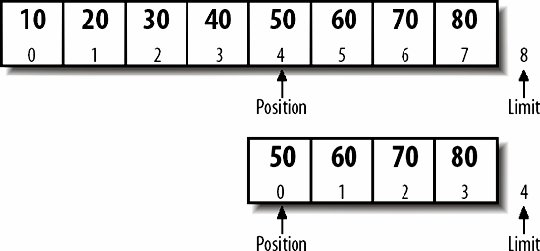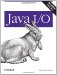# Slicing

A slice is similar to a duplicate. However, rather than including a complete copy of the original's data, it includes only a subsequence. This subsequence begins at the original's position when the slice is made and continues until the original's limit. Because the data is shared, changes to the elements in the original buffer also change the slice, and vice versa. However, the position, limit, and mark in the slice are all independent of the position, limit, and mark in the original. The slice's capacity will be less than or equal to the original's capacity. Furthermore, they index differently. Position 5 in the original might be position 0 in the slice, in which case position 6 in the original is position 1 in the slice, position 7 is position 2, and so forth.

For example, suppose we put 8 multiples of 10 in an IntBuffer, like so:

```IntBuffer original = IntBuffer.allocate(8);
original.put(10).put(20).put(30).put(40).put(50).put(60).put(70).put(80);
```

The original buffer is now in the state shown in Figure 14-18.

Figure 14-18. A filled int bufferNow suppose we set the position to 4 and take a slice:

```original.position(4);
IntBuffer slice = original.slice( );
```

Now we have two buffers, as shown in Figure 14-19. We can get from either one without changing the other. However, putting in the slice or putting in the original from position 4 on will affect the other buffer.

Slices are often useful for chopping headers off data. For example, a PNG image consists of an initial 8-byte signature (in hexadecimal), 0x89 0x50 0x4E 0x47 0x0D 0x0A 0x1A 0x0A, followed by three or more chunks of data. Each chunk consists of four parts:

Figure 14-19. A buffer and a slice of the buffer1. A 4-byte big-endian integer giving the length of the data in the chunk. Although unsigned, the value is between 0 and 231-1.
2. A 4-byte ASCII signature such as IHDR or tIME identifying the type of the chunk.
3. The chunk data with the length given by field 1. This can be empty. (That is, its length can be 0.)
4. A 4-byte CRC checksum for the chunk. The checksum is calculated over fields 2 and 3; that is, the signature and the data but not the length.

Let us suppose that you have memory mapped the entire contents of a PNG image into a read-only buffer named pngBuffer. The first thing you might do is chop off the 8-byte signature, which is constant and therefore uninteresting. Slicing accomplishes this:

```pngBuffer.position(8);
pngBuffer = buffer.slice( );
```

You might then wish to create separate buffers for each individual chunk of the PNG image. These separate buffers can be implemented by slicing the buffer at the beginning of each chunk's data and then setting the limit of the slice to the end of the data. For example, this code fragment will map the first such chunk's data:

```int i1 = buffer.get( );
int i2 = buffer.get( );
int i3 = buffer.get( );
int i4 = buffer.get( );
int size = i1 << 24 | i2 << 16 | i3 << 8 | i4
StringBuffer signature = new StringBuffer(4);
signature.append((char) buffer.get( ));
signature.append((char) buffer.get( ));
signature.append((char) buffer.get( ));
signature.append((char) buffer.get( ));
ByteBuffer firstChunkData = buffer.slice( );
firstChunkData.limit(size);
```

Subsequent chunks can be sliced similarly.Java I/O
ISBN: 0596527500
EAN: 2147483647
Year: 2004
Pages: 244